## Facebook Hacker Cup qualification round problem analysis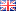Last weekend the qualification round for the 2012 Facebook Hacker Cup was held. Contestants had all weekend to solve at least one from a set of three problems in order to qualify. It turned out two of those were fairly easy, and the last one was pretty hard. I'll address the problems in order of increasing difficulty. If you want to try to solve these problems on your own, stop reading now, because there are spoilers ahead!

#### Alphabet Soup

Problem description.

The last problem in the set was actually the easiest of the three. There are several different ways to approach the problem and with at most a 1000 characters per test case it would be hard to design a solution that doesn't run in time.

For each letter, we can count how often it occurs in the source text and how often it occurs in the target word ("HACKERCUP" for this problem). The quotient of the two is an upper bound on how many times we can reconstruct the target word. After all, to spell "HACKERCUP" x times , we need x H's, x A's, 2x C's (since the letter C occurs twice in "HACKERCUP"), et cetera. Note that we need to round down since we can't use half a letter to spell half a word.

A straightforward solution in Python:
Python:
 ```1 2 3 4 5 6 7 8 9 10 ``` ```def solve(line): goal = "HACKERCUP" return min(line.count(ch)//goal.count(ch) for ch in goal) # Input/output from sys import stdin T = int(stdin.readline()) for t in range(T): line = stdin.readline() print('Case #%d: %d'%(t + 1, solve(line)))```

#### Billboards

Problem description.

For this problem we are asked to figure out the largest font size at which a given text still fits on a billboard of fixed dimensions. The tricky part is that we must layout the text ourselves, wrapping lines at word boundaries.

The simplest approach is to try increasing font sizes until the text no longer fits. In principle, binary search could be used to optimize this part of the solution, but with the given constraints that's not necessary.

That leaves the question of how to determine whether a certain text fits on the billboard. A nice trick is to not scale up the text, but to scale down the billboard and pretend our font always needs exactly 1 unit of length per letter. Then, we simply try to put as many words on each line as possible, truncating lines if necessary to end on a word boundary.

Python:
 ```1 2 3 4 5 6 7 8 9 10 11 12 13 14 15 16 17 18 19 20 21 22 ``` ```def fits(W, H, L): 'Returns whether text L fits on a W by H billboard.' pos = 0 for line in range(H): pos += W if pos >= len(L): return True pos = L.rfind(' ', 0, pos + 1) + 1 return False def solve(W, H, L): size = 0 while fits(W//(size + 1), H//(size + 1), L): size = size + 1 return size # Input/output from sys import stdin T = int(stdin.readline()) for t in range(T): W, H, L = stdin.readline().strip().split(None, 2) print('Case #%d: %d'%(t + 1, solve(int(W), int(H), L)))```

There are probably a few different ways to write the fits() function that achieve the same result. I chose to use the built-in rfind() function to align the cursor position to the last word boundary. This requires careful indexing to end up at the right location (specifically, we want to place the cursor at the first character after the last space).

#### Auction

Problem description.

The last problem was very hard, even by programming competition standards, due to the large numbers in the test data. Less experienced participants might be tempted to explicitly generate all pairs of prices and weights for all products, and then compare them pairwise, yielding a solution algorithm that runs in quadratic time.

Although that approach works for the sample data, the maximum possible number of products in the real test data is extremely large (up to N=1018, or a billion times a billion!) and such a solution will not finish in time. In fact, N is so large that even a linear-time (O(N)) algorithm will not run in time.

Before we return to efficiency concerns, let's first get a better idea of what good and bad deals look like, by plotting a number of points on 2D graph: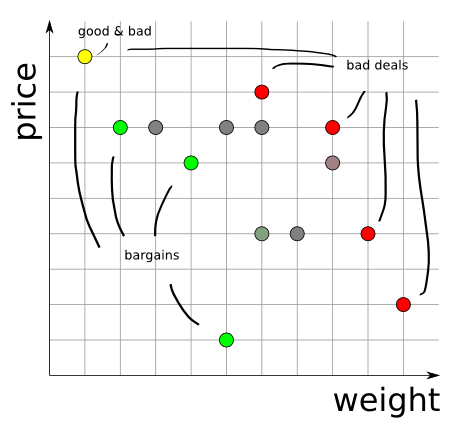As the graph shows, good deals decrease in weight as they increase in price. Similarly, bad deals decrease in weight as they increase in price. This is useful information because it means that if we have a list of products ordered by increasing prices, we only need to go through this list once to determine which are the good deals: exactly those products that have lower weight than the last good deal found. (And similarly for the bad deals, but going through the list in reverse order.)

Another useful observation is that for a fixed price point (a horizontal line in the graph) only the product with the lowest weight (i.e. the leftmost point in the graph) can possibly be a "bargain". Similarly, only the product with the highest weight (the rightmost point) can be a "terrible deal". Sometimes these points coincide: look at the yellow dot on the top left.

This hints at a possible solution approach. Due to the way prices are generated, there are at most 107 different price points, and since we only need to know the minimum and maximum possible weight at that price point, we only need to consider 2×107 different price & weight pairs. However, for each price & weight pair, we must also determine how many products exist with those exact properties.

To solve this problem efficiently, we need to exploit the fact that the weights and prices of the products are generated by a pair of linear congruential random number generators. As is the case with all pseudo-random number generators, their output is eventually periodic. Since the only state used by a linear-congruential generator is the previously generated value, and these values are in range 1 to M (for prices) or 1 to K (for weights) we can deduce that the generated sequence of prices will become periodic after less than M iterations, and will have a period of at most M (and similarly for weights).

Since M (and K) are relatively small we can afford to first peel off the non-periodic part of the generated sequences and then generate an entire period for weights and prices independently. Unfortunately, the periods for weights and prices are likely to be distinct and many not even have a divisor greater than 1 in common, which means that there are potentially near 1014 distinct price & weight pairs. Although this is much less than the maximum number of products (1018) it is still way too many to generate explicitly.

To solve this final part of the puzzle, consider that for most prices the sequence of corresponding weights is the same, although we enter the sequence at different positions.

This is best illustrated with a short example. Suppose the prices cycle over values [1,2] and the weights over values [3,4,5] and we generate eight products:

 product prices weight 1 2 3 4 5 6 7 8 1 2 1 2 1 2 1 2 3 4 5 3 4 5 3 4

The price & weight pairs cycle with a period of 6 (since 6 is the least common multiple of 2 and 3) which is why product 1 and 7 have the same properties, as do product 2 and 8. Now let's re-order that table by price:

 product prices weight 1 3 5 7 2 4 7 6 1 1 1 1 2 2 2 2 3 5 4 3 4 3 5 4

The weights for products with price=1 are 3,5,4,3 etc. and for price=2 they are 4,3,5,4. These sequences are the same, except that the starting weights (3 and 4, respectively) are different!

In this example N (the number of products) was greater than the combined period of the generated prices and weights, which means that the minimum and maximum weight are the same for each price. When N is less than that, that is generally not the case. For example, if we generate only 2 products, than the only weight at price=1 is 3, and the only weight at price=2 is 4.

We can use these observations to compute each (periodic) sequence of weights only once. Then, for every distinct price point, we only need to find the start and end point in the sequence of weights, figure out the mimimum and maximum weights in that range. The last operation requires some sort of index on the sequence of weights to work efficiently. A tree-based index can be used to solve range queries in logarithmic time, resulting in an O(M log K) algorithm, which is just fast enough for this problem.

To avoid making a long explanation even longer, I've glossed over some details; I'm sure readers that have been able to follow the explanation up to this point are able to fill in those details themselves.

For those who are curious how I implemented these details, I posted my solution (written in C++) to Pastebin. But beware: it's long and complicated! Source code for other solutions (submitted during the contest) is available from the Hacker Cup scoreboard.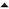01-'12 Facebook Hacker Cup round 1 problem analysis

I like the intent of the blog but, not being intimately familiar with the given problems, I feel there's something missing: the actual (and complete but maybe summarized) problem description.

Nice work!

I came out with nearly the same ideas about the auction-problem. (Unfortunately after trying an O(M*K) algorithm on the input-file... which took more than 6 mins)

But how do you solve the range queries for min/max in logarithmic time? I have an idea, but that uses O(K log K) memory. (Saving for every index the min/max of the following 1/2/4/8/16/... sized ranges, so that you can combine any r-length range in O(log r) ~ O(log K) steps)
I'm hoping for an data structure that uses only O(K) memory ... what are you using?

best regards
Thomas

Hey again,

I finally found the phrase to google for. "Range Minimum Query" (RMQ)
And actually there are some datastructures for the 1D-RMQ that can be build in O(K), use O(K) additional space and any range minimum query will take O(1).

That means, the total algorithm can be (theoretically... someone has to implement it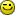) reduced to a total time complexity of O(M+K) ... jeaa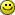best regards
Thomas

RobIII schreef op dinsdag 24 januari 2012 @ 02:26:
I like the intent of the blog but, not being intimately familiar with the given problems, I feel there's something missing: the actual (and complete but maybe summarized) problem description.
Good point; I've uploaded them to pastebin and linked them in the post.
Thomas schreef op dinsdag 24 januari 2012 @ 05:08:
But how do you solve the range queries for min/max in logarithmic time? I have an idea, but that uses O(K log K) memory. (Saving for every index the min/max of the following 1/2/4/8/16/... sized ranges, so that you can combine any r-length range in O(log r) ~ O(log K) steps)
That describes basically what I did (though I used an explicit tree structure).

You're right to note that this is far from optimal. It was just the first thing that came to mind to speed up the search and it seems good enough for this problem (even if just barely) though I agree that an O(K+M) solution would be even nicer!

I wrote up an alternative solution to the Billboard problem on my blog. I am in progress to post up another solution to Auctions problem.

Algorithm
So lets figure out the intelligent way.

1. The max word that can fit on any line is 1

2. The max number of lines that you can fit on the billboard is the number of words.

Presto!. That gives you the range of font sizes to work in.

1. Lets pick the bound of the font size. the min and max will be font size that you will require.

Pick the height of the bill board and divide by max length of a word in the given string of inputs.
Pick the width of the billboard and divide by max length of a word in the given string of inputs.
Pick the height and the width and divide by max number of words in the given string of inputs.
Worse case Lower Bound is still 1. I will deal with this later
2. Now iterate between the lower and upper bounds of the font size.
Add a word starting with the first one
Check the remaining width to add a text.
Attempt to add a second word to the string. if it fits continue adding words (dont forget the space between the words), if not then increment the number of line and continue from begining
Check if the number of lines times font size is less than the height of the billboard. If it fails pick the next font size, else continue.
Simple!!! No need to brute force everything from 1 to 1000. Bingo.

http://wp.me/p26UHS-1j

I'm still a bit puzzled after your explanation, perhaps you could clarify for me this;

Unfortunately, the periods for weights and prices are likely to be distinct and many not even have a divisor greater than 1 in common, which means that there are potentially near 1014 distinct price & weight pairs. Although this is much less than the maximum number of products (1018) it is still way too many to generate explicitly.

To solve this final part of the puzzle, consider that for most prices the sequence of corresponding weights is the same, although we enter the sequence at different positions
.

Am I correct in stating that the last part is an assumption? True for most inputs, but not all?
It was my belief that the worst-case scenario has no less than 1014 different options, which would leave you no choice as to handle all these options..

If that is true, than I don't think it's a very good challenge, as no one would be able to write a program which could handle all possible inputs in time.. ( given current hardware )

[Comment edited on dinsdag 24 januari 2012 15:23]

Arjan schreef op dinsdag 24 januari 2012 @ 15:23:
Am I correct in stating that the last part is an assumption? True for most inputs, but not all?
One of the details I glossed over was that the periods of the sequences are not necessarily coprime. If they are (as in my example) then nearly 1014 distinct pairs are possible (nearly but strictly less than 1014 because 107 is not coprime to itself).

If the sequences of prices and weights have periods P and Q respectively, then there are gcd(P,Q) different sequences of weights, each of length Q/gcd(P,Q). I glossed over this detail (by giving an example with P=2 and Q=3) so let's look at an example where prices cycle over six values [1,2,3,4,5,6] and weights over eight values [1,2,3,4,5,6,7,8]. In this scenario, even prices get paired to even weights only, and odd prices to odd weights. So there are two subsequences of weights: [1,7,5,3] for the odd prices, and [2,8,6,4] for the even prices.

So what I hinted at with "most" (and which I indeed did not explain very clearly) is that either you have a single sequence with period near 107 when gcd(P,Q)=1 (and this is actually the worst case from a complexity point of view) or you have multiple shorter ones which combine to the same total length. In either case, the solution I outlined can solve even the most difficult cases in a reasonable amount of time.

Nice Work,
but how come you post the code to all tasks but the last one? Wouldn't everybody who was able to solve that task not only in theory, but also programmatically be more than glad to share it?

Post it or it didn't happen!

You're right, Felix: I didn't post the source code yet because my solution was so contrived I was not 100% sure it was correct. Since there is already too much misinformation on the Internet, I waited with posting the solution until I had a chance to validate it. Since it turns out it worked perfectly, I've added a link to the source code.

Does anyone have a sample test input with same output? I want to code the solution to the auction problem and I want to make sure I properly figure it out.

Thanks.

Very good Blog Post.
And congrats on solving Auction. I myself gave up after clearing the other two Problems. I wish you much succes for the coming rounds.
Benno

[quote]Sam schreef op dinsdag 24 januari 2012 @ 14:11:

1. The max word that can fit on any line is 1

2. The max number of lines that you can fit on the billboard is the number of words.

Presto!. That gives you the range of font sizes to work in.
[/q

You can optimize even more if you start the search not from the minimum (or the maximum) font size in the range, but to take into account the W / H ratio of particular billboard.